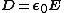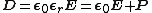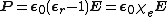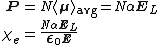# electric susceptibility

Also found in: Dictionary, Thesaurus, Medical, Wikipedia.

## Electric susceptibility

A dimensionless parameter measuring the ease of polarization of a dielectric. In a vacuum, the electric flux density D (measured in coulombs/m2) and electric field strength E (volts/m) are related by Eq. (1) where

(1)ε0 is the permittivity of free space, having the value 8.854 × 10-12 farad/m. In a dielectric material, polarization occurs, and the flux density has the value given by Eq. (2),
(2)where εr is the relative permittivity of the material and P is the polarization flux density. This can be written as Eq. (3), where &khgr;e =
(3)εr - 1 is known as the electric susceptibility of the dielectric material. It is a measure of that part of the relative permittivity which is due to the material itself.

The electric susceptibility can be related to the polarizability α by expressing the polarization in terms of molecular parameters. Thus Eqs. (4)

(4)hold, where N is the number of molecules per unit volume, 〈 μ avg〉 is their average dipole moment, and E L is the local electric field strength at a molecular site. At low concentrations, E L approaches E , and the susceptibility is proportional to the concentration N. For a discussion of the properties and measurement of electric susceptibility See Permittivity, Polarization of dielectrics

McGraw-Hill Concise Encyclopedia of Physics. © 2002 by The McGraw-Hill Companies, Inc.

## electric susceptibility

[i¦lek·trik sə‚sep·tə′bil·əd·ē]
(electricity)
A dimensionless parameter measuring the ease of polarization of a dielectric, equal (in meter-kilogram-second units) to the ratio of the polarization to the product of the electric field strength and the vacuum permittivity. Also known as dielectric susceptibility.
McGraw-Hill Dictionary of Scientific & Technical Terms, 6E, Copyright © 2003 by The McGraw-Hill Companies, Inc.
Site: Follow: Share:
Open / Close